# Addition, Subtraction, Multiplication, DivisionPage 1

#### WATCH ALL SLIDES

Slide 1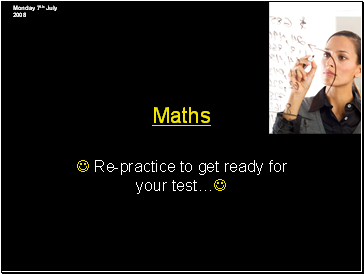## Maths

Monday 7th July 2008

Slide 2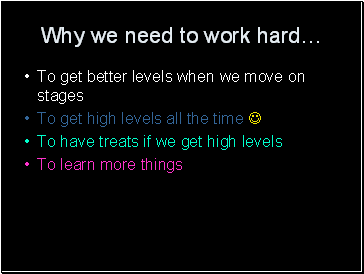## Why we need to work hard…

To get better levels when we move on stages

To get high levels all the time 

To have treats if we get high levels

Slide 3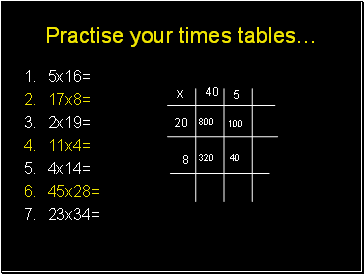5x16=

17x8=

2x19=

11x4=

4x14=

45x28=

23x34=

x

Slide 4## Practise taking away…

54-12=

998-257=

555-321=

783-194=

513-427=

671-462=

482-123=

Slide 5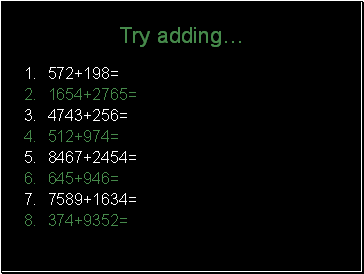572+198=

1654+2765=

4743+256=

512+974=

8467+2454=

645+946=

7589+1634=

374+9352=

Slide 6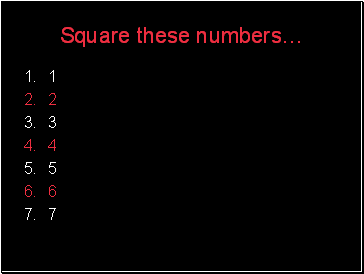## Square these numbers…

1

2

3

4

5

6

7

Slide 7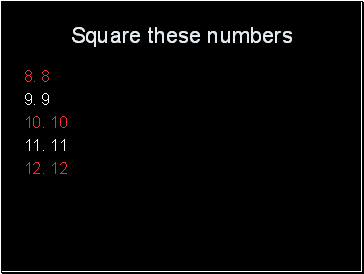Square these numbers

8. 8

9. 9

10. 10

11. 11

12. 12